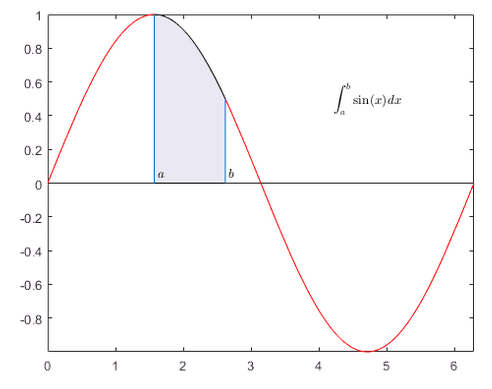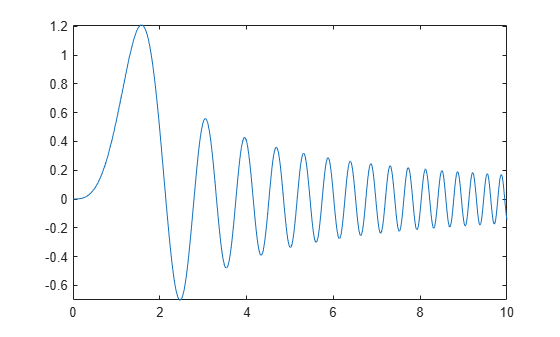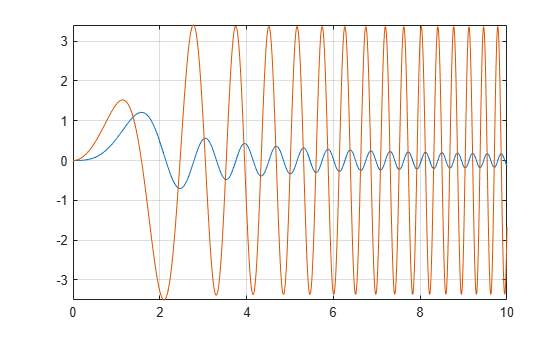## Integration

This example shows how to compute definite integrals using Symbolic Math Toolbox™.### Definite Integral

Show that the definite integral $\underset{a}{\overset{b}{\int }}f\left(x\right)dx$ for $f\left(x\right)=sin\left(x\right)$ on $\left[\frac{\pi }{2},\frac{3\pi }{2}\right]$ is 0.

```syms x int(sin(x),pi/2,3*pi/2)```
`ans = $0$`

### Definite Integrals in Maxima and Minima

To maximize $F\left(a\right)={\int }_{-a}^{a}\mathrm{sin}\left(ax\right)\mathrm{sin}\left(x/a\right)\phantom{\rule{0.16666666666666666em}{0ex}}dx$ for $a\ge 0$, first, define the symbolic variables and assume that $a\ge 0$:

```syms a x assume(a >= 0);```

Then, define the function to maximize:

`F = int(sin(a*x)*sin(x/a),x,-a,a)`
```F =  ```

Note the special case here for $a=1$. To make computations easier, use `assumeAlso` to ignore this possibility (and later check that $a=1$ is not the maximum):

```assumeAlso(a ~= 1); F = int(sin(a*x)*sin(x/a),x,-a,a)```
```F =  $\frac{2 a \left(\mathrm{sin}\left({a}^{2}\right) \mathrm{cos}\left(1\right)-{a}^{2} \mathrm{cos}\left({a}^{2}\right) \mathrm{sin}\left(1\right)\right)}{{a}^{4}-1}$```

Create a plot of $F$ to check its shape:

`fplot(F,[0 10])`Use `diff` to find the derivative of $F$ with respect to $a$:

`Fa = diff(F,a)`
```Fa =  ```

The zeros of $Fa$ are the local extrema of $F$:

```hold on fplot(Fa,[0 10]) grid on```The maximum is between 1 and 2. Use `vpasolve` to find an approximation of the zero of $Fa$ in this interval:

`a_max = vpasolve(Fa,a,[1,2])`
`a_max = $1.5782881585233198075558845180583$`

Use `subs` to get the maximal value of the integral:

`F_max = subs(F,a,a_max)`
`F_max = $0.36730152527504169588661811770092 \mathrm{cos}\left(1\right)+1.2020566879911789986062956284113 \mathrm{sin}\left(1\right)$`

The result still contains exact numbers $\mathrm{sin}\left(1\right)$ and $\mathrm{cos}\left(1\right)$. Use `vpa` to replace these by numerical approximations:

`vpa(F_max)`
`ans = $1.2099496860938456039155811226054$`

Check that the excluded case $a=1$ does not result in a larger value:

`vpa(int(sin(x)*sin(x),x,-1,1))`
`ans = $0.54535128658715915230199006704413$`

### Multiple Integration

Numerical integration over higher dimensional areas has special functions:

`integral2(@(x,y) x.^2-y.^2,0,1,0,1)`
```ans = 0 ```

There are no such special functions for higher-dimensional symbolic integration. Use nested one-dimensional integrals instead:

```syms x y int(int(x^2-y^2,y,0,1),x,0,1)```
`ans = $0$`

### Line Integrals

Define a vector field `F` in 3D space:

```syms x y z F(x,y,z) = [x^2*y*z, x*y, 2*y*z];```

Next, define a curve:

```syms t ux(t) = sin(t); uy(t) = t^2-t; uz(t) = t;```

The line integral of `F` along the curve `u` is defined as $\int f\cdot du=\int f\left(ux\left(t\right),uy\left(t\right),uz\left(t\right)\right)\cdot \frac{du}{dt}\phantom{\rule{0.16666666666666666em}{0ex}}dt$, where the $\cdot$ on the right-hand-side denotes a scalar product.

Use this definition to compute the line integral for $t$ from $\left[0,1\right]$

`F_int = int(F(ux,uy,uz)*diff([ux;uy;uz],t),t,0,1)`
```F_int =  $\frac{19 \mathrm{cos}\left(1\right)}{4}-\frac{\mathrm{cos}\left(3\right)}{108}-12 \mathrm{sin}\left(1\right)+\frac{\mathrm{sin}\left(3\right)}{27}+\frac{395}{54}$```

Get a numerical approximation of this exact result:

`vpa(F_int)`
`ans = $-0.20200778585035447453044423341349$`

#### Mathematical Modeling with Symbolic Math Toolbox

Get examples and videos